Published: 31 December 2017

# Vibration modal shapes and strain measurement of the main shaft assembly of a friction hoist

Chi Ma1
Lulu Zhang2
Congwang Bao3
Yuqiang Jiang4
Xingming Xiao5
1, 2, 3, 4, 5School of Mechanical and Electrical Engineering, China University of Mining and Technology, Xuzhou, China
1, 4School of Information and Control Engineering, China University of Mining and Technology, Xuzhou, China
Corresponding Author:
Xingming Xiao
Views 153

#### Abstract

In order to evaluate the reliability of the main shaft unit of a friction hoisting system, strain measurement is a significant method. In this paper, a test rig of a friction hoisting system was built, which could applied periodically changing load on its main shaft unit; The mechanical analysis under the test load was conducted and the boundary limits were obtained; A three dimensional model of the main shaft unit was built in Pro-E and its finite element analysis was performed in ANSYS; With the analytical result, measuring points for strain rosettes were initially selected; Vibration modal shapes of the main shaft unit were analyzed, based on which Modal Assurance Criterion (MAC) was utilized in the Particle Swarm Optimization (PSO) algorithm to make the final decision of the number and positions of the measuring points; A wireless measurement system was developed to acquire strain signals from the optimized measuring positions; The test result verified the efficiency of the methods employed in this paper and revealed how strain of the main shaft unit changes during running process.

## 1. Introduction

Multi-rope friction hoists are widely used in coal mines. It’s of great significance to ensure that they work in a continuous and reliable condition. Hence, a lot of research on the examination of the performance of these hoists have been carried out. Topics like brake performance, hoisting load, vibration of bearing and gears attract a great deal of research enthusiasm . Relatively speaking, not so much study has been put on the stress or strain of the mail shaft unit, which includes drum, shaft and wheel spoke. However, with the development of computer science and the emergence of powerful analytical software, relevant research has been growing fast.

The main shaft unit is a crucial component for a friction hoist to transfer power and bear load. Its dynamical behavior is related to the healthy status of a hoist. As an indirect way, vibration analysis is usually used to investigate shaft bend, imbalance, component loosing, and bearing defect. When it comes to the stress or potential problems related to strength or rigidity, the direct way, strain measurement works better. Strain of the main shaft unit is caused by the torque acted upon the joint between shaft and motor as well as the pressure from steel wire ropes. Change of hoisting load can be easily tracked based on the analysis on the strain of main shaft unit.

There have been some fruitful research on the strain or stress of main shaft unit: Jing Li  performed a stress analysis under different operating conditions which include steady hoisting, left drum start, left drum stop, right drum start and right drum stop, and simulated the stress-strain field numerically for the first three conditions. Ying Wang  and Junhong You  utilized ANSYS to analyze the deformation regularity, deflection change and stress concentration, and verified the position of the maximum stress, regularity of deflection change and regularity of stress wave. Li  constructed a finite element model for a cast head sheave and analyzed the stress distribution of a wheel spoke and flange. Wolny  investigated stress distribution of cylindrical shell and wheel spoke and analyzed the most dangerous stress points.

Among current relevant research, theoretical analysis and finite element simulation take up a large proportion. Because of many influence factors, obvious deviation still exists between the simulation result and the practice. Furthermore, lack of optimization for strain measuring points makes it difficult to achieve the best testing effect with limited measurement resources.

On the basis of current research achievement and aiming at their inadequacy, this paper involves stress and strain simulation, measurement point optimization, and construction of an actual measurement system, which will largely benefit the reliability of the main shaft unit.

## 2. Test rig

In order not to affect regular coal production, a test rig shown as Fig. 1was constructed, which was an equivalent transformation from actual hoisting systems. Its components include hydraulic brake station, main shaft unit, brake, steel wire ropes, sheave frame, sheave groups, balance cylinders, operating desk, adjusting screws and ground bolt.

Fig. 1Design of test rig: 1 – operation desk, 2 – sydraulic brake station, 3 – main shaft unit, 4 –brake, 5 – Foundation bolt, 6 – steel wire ropes, 7 – sheave frame, 8 – sheave groups, 9 – balance cylinder, 10 – adjusting screw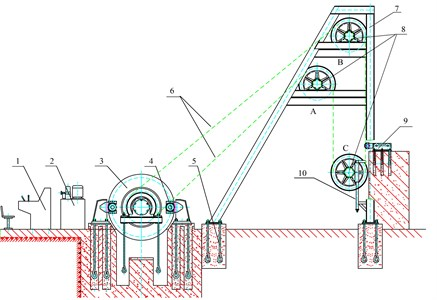Fig. 2Structure of main shaft unit of the test rig: 1 – main shaft, 2 – hollow cover, 3 – bearing block, 4 – self-aligning roller bearing, 5 – friction drum, 6 – friction liner, 7 – lock block, 8 – brake disc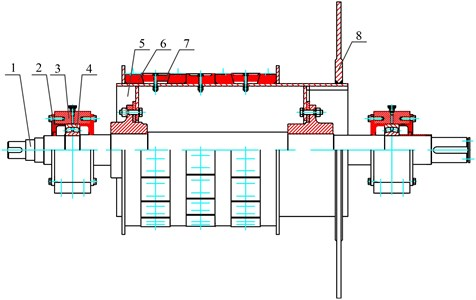The main shaft unit is the research object of this paper. It’s mainly made up of a main shaft, supporting bearings, a friction drum and friction liners. The detailed structure of the main shaft unit is shown as Fig. 2. Because the main shaft unit is a rotating assembly, collection of its strain signals is inconvenient as usual and cannot be conducted through traditional ways. Generally, collector ring and wireless communication are typical ways to deal with this issue. Collector ring is more traditional than wireless communication. It has some defects. For example, the accuracy is sometimes severely affected by the virtual strain caused by collector ring or breakpoints between copper ring and wire. Hence, according to the principle of measurement accuracy, reliable installation, convenient dismounting and no destruction to original structure and performance, wireless communication was finally utilized to acquire the stress signals of friction drum and main shaft unit. Fig. 3 shows the principle of the wireless signal acquiring system developed in this paper. Strain rosettes were pasted at relevant positions inside the friction drum and on the main shaft to realize stress measurement [7-9]. Strain signals were transmitted by the wireless signal collector, then received by the wireless receiver, and finally reached the industrial PC through network cables.

Fig. 3Strain testing system of main shaft device: 1 – main shaft unit, 2 – wireless signal collector, 3 – strain rosette, 4 – wireless receiver, 5 – network cable, 6 – industrial PC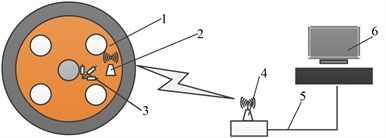Quantity and positions of the strain rosettes determine both the cost and the effect of strain measurement, so there is great necessity and significance in optimization of the quantity and positions of measuring points. An ideal optimization should achieve the balance between measurement cost and effect. Proper arrangement of measuring points facilitates the detection of impact of each modal shape on stress of the main shaft unit, and in the meantime, current measurement resource such as signal sampling channels should be fully utilized.

## 3. Optimization of strain measuring points

### 3.1. Optimizing scheme

How to use the limited measurement resource to achieve an ideal measurement as accurate as possible? It’s necessary to make an optimization of strain measuring points. According to different measurement demands, current frequently used optimizing methods include ordination, nonlinear programming, random cluster and reckoning. Based on the actual conditions of the main shaft unit, the following scheme shown as Fig. 4 was adopted : a mechanical model was first constructed with analytical method to get the boundary limits, then a three dimensional model built in Pro-E was imported into ANSYS to perform a stress simulation for the initial selection of measuring points. Modal shapes were obtained with modal analysis, and finally with the max nondiagonal element as the fitness value, an optimizing algorithm based on MAC and PSO was designed.

Fig. 4Optimization flowchart of measuring points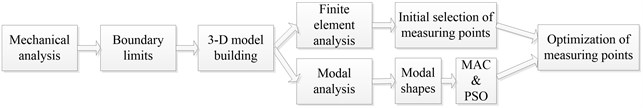### 3.2. Mechanical analysis

The test rig uses a floor standing friction drum. The segment of ropes that winds round the drum has two ends, one is tight and the other one is loose. As shown in Fig. 5, tensions at the two ends are ${F}_{1}$ and ${F}_{2}$. The rope arc round the drum is divided into static arc and slip arc. The central angle of slip arc is ${\alpha }_{R}$, and the rest part is static arc whose central angle is $\alpha$-${\alpha }_{R}$. The friction force transferred by the drum is:

1
$F={F}_{2}\left({e}^{\mu {\alpha }_{R}}-1\right),$

where $\mu$ is the friction coefficient between the ropes and the drum, and $e$ is the Napierian base.

In static arc, the drum is under both a tangential and a radial force while there is only radical force in slip arc. The tangential and radial force at central angle $\theta$ on the drum is:

2
${F}_{t\theta }=\left\{\begin{array}{l}\frac{2×{\mu F}_{2}{e}^{\mu \theta }}{BD},\left(0\le \theta \le {\alpha }_{R}\right),\\ 0,\left({\alpha }_{R}\le \theta \le 2\pi \right),\end{array}\right\$
3
$\left\{\begin{array}{l}\frac{2×{F}_{2}{e}^{\mu \theta }}{BD},\left(0\le \theta \le {\alpha }_{R}\right),\\ 0,\left({\alpha }_{R}\le \theta \le \pi \right),\end{array}\right\$

where $D$ is the dimeter of the drum, and $B$ is the contact width between drum and liners.

Fig. 5Tension analysis of friction drum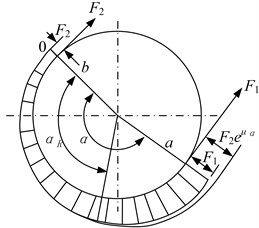Main parameters of the test rig are listed in Table 1.

Table 1Main parameters of main shaft

 Diameter of the drum (mm) 800 Max tension of ropes (kN) 15 Diameter of head sheave (mm) 800 Max hoisting velocity (m/s) 1.65 Surrounding angle (°) 180 Diameter of rope (mm) 12 Quantity of ropes 4 Space between ropes (mm) 250 Friction coefficient of the liner 0.25 Width of liner (mm) 94

When the tension at tight end is increased to the maximum value which is 15 kN here, all the surrounding arc becomes slip arc, and the tension at loose end is ${F}_{2}=$ 6.8 kN. With Eq. (2) and Eq. (3), the tangential ${F}_{t\theta }$ and radial force ${F}_{r\theta }$ on the drum can be calculated:

4
${F}_{t\theta }=\left\{\begin{array}{l}0.18×{e}^{0.25×\theta },\left(0\le \theta \le \pi \right),\\ 0,\left(\pi \le \theta \le 2\pi \right),\end{array}\right\$
5
${F}_{r\theta }=\left\{\begin{array}{l}0.045×{e}^{0.25×\theta },\left(0\le \theta \le \pi \right),\\ 0,\left(\pi \le \theta \le 2\pi \right).\end{array}\right\$

### 3.3. Finite element analysis

With the above boundary load, finite element analysis on the main shaft unit was performed [11, 12]. The main shaft is actually an assembly of many parts, but regarded as a solid during the finite element analysis. The first step of this analysis was to build a three dimensional model in Pro-E, which was saved in the file format of “parasolid” and then imported into ANSYS.

The next step was model meshing. The quantity of mesh quantity affects both precision and efficiency of simulation. Algebraic generation method was adopt in this paper. More detailed meshing was performed where needed, such as the places near screwed holes. In this case, the total numbers of the units and nodes were 67275 and 271923, respectively.

When the load was applied on the model of the main shaft unit, its stress contour was obtained, as shown in Fig. 6. From this contour, it can be found that the max stress point is near the joint between the bearing support and the motor. The maximum stress is 91.41 MPa, much lower than the yield limit of the material 45 Mn, 375 MPa.

Then primary selection of measuring points could be made, on which pasting convenience should be taken into account. Inner wall, wheel spoke and the shaft segment near the brake disk were chosen as measurement positions, and the exact measuring points initially selected are shown as Fig. 7.

Fig. 6Stress contour of the main shaft unit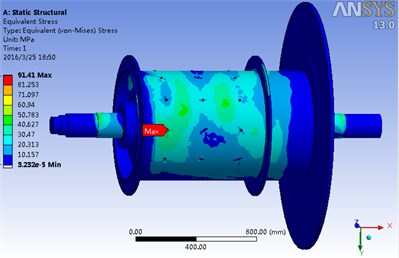Fig. 7Primary selected measuring points of the main shaft unit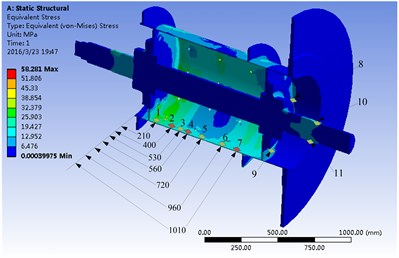### 3.4. Optimization of measuring points

Optimization criterion affects the result significantly. Effective Independent Evaluation Criterion (EI) and Modal Assurance Criterion (MAC) [13-15] are frequently adopted. In consideration of searching efficiency, MAC was employed in this paper.

Ideal modal shapes are linearly independent one another, but various noise decreases their orthogonality obviously. Fortunately, when proper quantity and position of test points are achieved, spatial angle between modal shapes can be kept large enough. Array of MAC reflects the spatial angle and is a significant index for the evaluation of the correlation among modal vectors. An element of MAC array is calculated by:

6
${MAC}_{ij}=\frac{{\left({\mathrm{\varnothing }}_{i}^{T}{\mathrm{\varnothing }}_{j}\right)}^{2}}{{\left(\mathrm{\varnothing }}_{i}^{T}{\mathrm{\varnothing }}_{j}\right)\left({\mathrm{\varnothing }}_{j}^{T}{\mathrm{\varnothing }}_{i}\right)},$

where ${\mathrm{\varnothing }}_{j}$ and ${\mathrm{\varnothing }}_{j}$ are the modal vectors of order $i$ and $j$. Elements of MAC array are between 0 and 1. The bigger an element is, the smaller the spatial angle is, and the element of value 0 means no correlation while 1 means complete correlation. Furthermore, the smaller the nondiagonal elements are, the more appropriate the placement of measuring points is.

A modal analysis [17-19]of the finite model of the main shaft unit was made with fixed constrains applied on the places of two bearing and the solving order set as 8. The first 8 natural frequencies and shapes are listed in Table 2 and shown as Fig. 8.

Table 2First 8 natural frequencies and modes of the main shaft unit

 Order Frequency (Hz) Modal shape Order Frequency (Hz) Modal shape 1 82.382 Axial twist 5 218.37 Horizontal swing 2 160.72 Horizontal swing 6 381.54 Horizontal swing 3 172.40 Vertical swing 7 415.53 Horizontal swing 4 206.92 Vertical swing 8 596.52 In-out swing

Fig. 8Modal shapes of the first 8 orders: a) modal shape of order 1, b) modal shape of order 2, c) modal shape of order 3, d) modal shape of order 4, e) modal shape of order 5, f) modal shape of order 6, g) modal shape of order 7, h) modal shape of order 8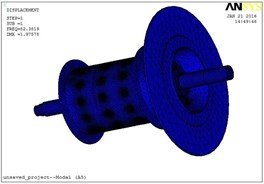a)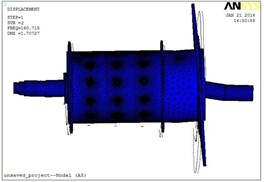b)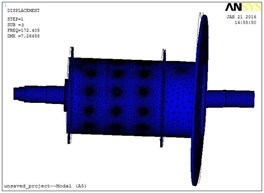c)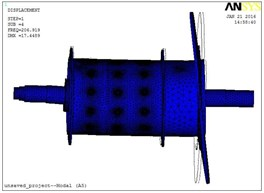d)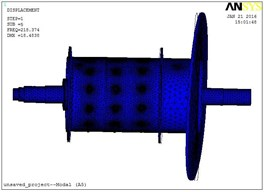e)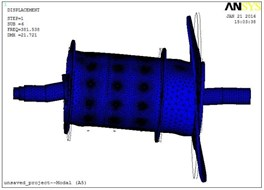f)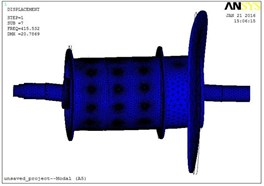g)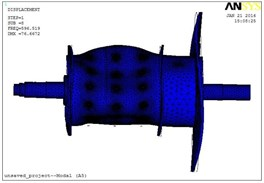h)

As listed in Table 2, natural frequency of the first order is 78.27 Hz, its modal shape is axial twist; The second natural frequency is 84.431 Hz, with a modal shape of horizontal swing; The third 137 Hz, vertical swing; the forth 142.16 Hz, vertical swing. For the following order, besides modal shapes listed in Table 2, some other modal shapes still exist. As shown in Fig. 8, the modal shape of the eighth order is an in-out swing which looks like shrinkage and expansion of the drum. According to this simulation result, total displacement modes of the initially selected test points are listed in Table 3.

Particle Swarm Optimization Algorithm (PSO) [20-23] was adopted in this optimization. PSO is an optimization method based on population search, which generates an optimal group from the original group iteratively. During this iterative process, quality of each new group should be evaluated. In this case, the max nondiagonal element of MAC array was taken as the fitness function. Smaller value of it means a better selection of test points. Other parameters of PSO algorithm are listed in Table 4.

Table 3The total displacement modes of the first 8 orders of the main shaft unit

 Point node Order 1 Order 2 Order 3 Order 4 Order 5 Order 6 Order 7 Order 8 1 31940 0.73439 0.32729 1.5775 2.7681 3.2046 12.458 14.332 5.0548 2 27686 0.74983 0.43857 1.6431 2.6166 3.4020 10.344 12.225 18.169 3 31524 0.75870 0.47936 2.0219 3.0296 3.8115 9.6790 11.280 31.561 4 24175 0.78236 0.59605 2.2730 3.0824 4.0255 7.8273 9.5641 45.881 5 31112 0.79270 0.64811 2.5935 3.1522 4.2552 5.3127 6.9241 42.316 6 20599 0.81233 0.73478 2.8316 3.0108 3.9726 4.5318 5.3775 34.613 7 25736 0.74003 0.38603 1.5796 2.6556 2.7942 11.272 11.471 1.528 8 33581 0.70657 0.85140 3.3179 2.4766 3.1995 3.4086 3.9959 1.5698 9 33614 0.71539 0.87200 3.2071 1.9619 3.8306 2.6603 4.7686 2.4925 10 42309 0.10936 0.47163 1.8838 0.84462 1.0674 2.4477 3.5743 0.84205 11 47892 0.07846 0.29146 1.4917 0.41090 0.88916 1.8792 2.7359 0.76842

Table 4Parameters of PSO algorithm

 Swarm scale Swarm range Velocity range Swarm dimensionality Acceleration constant Inertia weight Iteration number 20 [1, 165] [–0.5, 0.5] 8 2 0.927 40

Final optimization result was listed in Table 5.

Table 5Test point optimization

 Number of points Fitness value Point combination Number of points Fitness value Point combination 2 0.171 6, 9 7 0.1559 1, 6, 7, 8, 9, 10, 11 3 0.154 6, 9, 10 8 0.2096 1, 5, 6, 7, 8, 9, 10, 11 4 0.151 6, 9, 10, 11 9 0.2828 1, 2, 5, 6, 7, 8, 9, 10, 11 5 0.151 1, 6, 9, 10, 11 10 0.3642 1, 2, 4, 5, 6, 7, 8, 9, 10, 11 6 0.1518 1, 6, 8, 9, 10, 11 11 0.4409 1-11

When the fitness value is smaller than 0.25, modes are easily identified, and the point combination is better. As shown in Table 5, for number of points less than 9, the fitness value is smaller than 0.25, and the value for number 9 is 0.2828, which is fairly close to 0.25. In consideration of the available resource of the test channels, points 1, 5, 6, 7, 8, 9, 10 and 11 were chosen as the final measurement positions, among which the first six positions are marked in Fig. 9.

Fig. 9Optimized positions for strain rosettes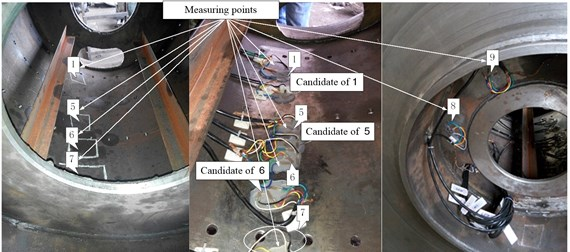## 4. Result

After strain rosettes were pasted, the wireless measurement system was built, and signals of strain were acquired. For the strain of the ninth point, strain signal was shown as Fig. 10, which contain strain of circumferencial direction and the direction of 45°. This signal was generated during a process which contained the start, running and stop operation of the test rig. It can be found that circumferencial strain of the wheel spoke fluctuates periodically within a range from –50 με to 100 με, which is not very intense; and at the stop moment, an obvious impact happens. For the other measuring positions, there are similar phenomenon.

Fig. 10Strain of the ninth measuring point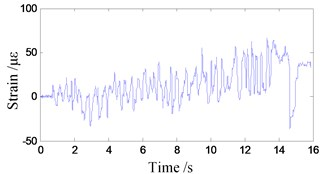a) Circumferencial strain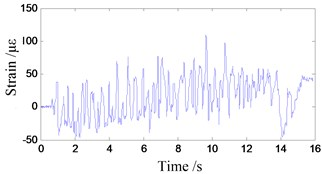b) Strain along direction 45°

## 5. Conclusions

Strain change of the main shaft unit during running is a key parameter to evaluate the performance of a friction hoisting system. In this paper, a test rig was built, with which a wireless measurement system was constructed to investigate the strain change. In order to make a right decision of the measuring points, mechanical analysis of the drum which is the main part of a main shaft unit was achieved, and the boundary limit was obtained. After a three dimensional model was built in Pro-E, finite element analysis of the main shaft unit was conducted in ANSYS. According to the analytical result, measuring points were initially selected. Vibration modal shapes of the main shaft unit were analyzed, based on which MAC was utilized in the Particle Swarm Optimization (PSO) algorithm to make the final decision of the number and positions of the measuring points. Through the above investigation, the following conclusion can be made:

1) The wireless strain measurement system works efficiently to acquire strain signals from the main shaft unit of a friction hoisting system, which would be a scientific basis for design improvement or adjustment of hoisting load.

2) When the MAC criterion based on vibration modal shapes is utilized, PSO algorithm is capable of conducting an ideal optimization for the number and position of measuring points.

3) Strain of the main shaft assembly of a friction hoist fluctuates periodically during running process and has always an impact at the stop moment.

#### Cited by

Fault diagnosis of head sheaves based on vibration measurement and data mining method
Control of Machining of Axisymmetric Low-Rigidity Parts

05 May 2017
Accepted
25 October 2017
Published
31 December 2017
SUBJECTS
Modal analysis and applications
Keywords
friction hoist
main shaft unit
strain measurement
finite element analysis
particle swarm optimization
modal assurance criterion
Acknowledgements

This work was supported by National Natural Science Foundation of China (Grant No. 51675520), the Fundamental Research Funds for the Central Universities (Grant No. 2014QNB19) and A Project Funded by the Priority Academic Program Development of Jiangsu Higher Education Institutions. Doctor Yansong Ma provided much help on the construction of the wireless strain measurement system, which has contributed a lot to this paper. Sincere appreciation is extended to him, and also to the reviewers of this paper for their helpful comments.

Author Contributions

Chi Ma who is the organizer of this whole research and analyzed the dynamical behavior of the main shaft assembly; Lulu Zhang’s job was to collect reference materials of this topic and did the fundamental theoretical study; Congwang Bao designed and set up the test rig; Yuqiang Jiang processed the collected signals and the experimental data; Xingming Xiao, as the advisor of this research team, gave significant guidance during the research.